Vilaseca, F., Puig, J., Pèlach, M. À., El Mansouri, N.-E., Alcalà, M., Roux, J.-C., and Mutjé, P. (2011). "Study and modeling of the disintegration kinetics of coated paper," BioRes. 6(2), 1659-1669.

#### Abstract

The disintegration of recovered paper is the first operation in the preparation of recycled pulp. It is known that the defibering process follows a first order kinetics from which it is possible to obtain the disintegration kinetic constant (KD) by means of different ways. The disintegration constant can be obtained from the Somerville index results (%ISV) and from the dissipated energy per volume unit (SS). The %ISV is related to the quantity of non-defibrated paper, as a measure of the non-disintegrated fiber residual (percentage of flakes), which is expressed in disintegration time units. In this work, disintegration kinetics from recycled coated paper has been evaluated, working at 20 rev/s rotor speed and for different fiber consistency (6, 8, 10, 12, and 14%). The results showed that the values of experimental disintegration kinetic constant, KD, through the analysis of Somerville index, as function of time, increased with the disintegration consistency. Therefore, as consistency increased, the disintegration time was drastically reduced. The calculation of the disintegration kinetic constant (modeled KD), extracted from the Rayleigh’s dissipation function, showed a good correlation with the experimental values using the evolution of the Somerville index or with the dissipated energy.

STUDY AND MODELING OF THE DISINTEGRATION KINETICS OF COATED PAPER

Fabiola Vilaseca,a,* Josep Puig,a M. Àngels Pèlach,a Nour-Eddine El Mansouri,a Manuel Alcalà,Jean-Claude Roux,c and Pere Mutjé a

The disintegration of recovered paper is the first operation in the preparation of recycled pulp. It is known that the defibering process follows a first order kinetics from which it is possible to obtain the disintegration kinetic constant (KD) by means of different ways. The disintegration constant can be obtained from the Somerville index results (%ISV) and from the dissipated energy per volume unit (SS). The %ISV is related to the quantity of non-defibrated paper, as a measure of the non-disintegrated fiber residual (percentage of flakes), which is expressed in disintegration time units. In this work, disintegration kinetics from recycled coated paper has been evaluated, working at 20 rev/s rotor speed and for different fiber consistency (6, 8, 10, 12, and 14%). The results showed that the values of experimental disintegration kinetic constant, KD, through the analysis of Somerville index, as function of time, increased with the disintegration consistency. Therefore, as consistency increased, the disintegration time was drastically reduced. The calculation of the disintegration kinetic constant (modeled KD), extracted from the Rayleigh’s dissipation function, showed a good correlation with the experimental values using the evolution of the Somerville index or with the dissipated energy.

Keywords: Coated paper; Disintegration kinetics; Somerville index; Dissipated power; Rayleigh’s equation; Modeling

Contact information: a: LEPAMAP Group, Department of Chemical Engineering, University of Girona, C/ M. Aurèlia Capmany, 61, 17071 Girona (Spain) T. +34 972418400; b: PRODIS Group, Department of Organization, Business Management and Product Design, University of Girona, C/ M. Aurèlia Capmany, 61, 17071 Girona (Spain); c: Laboratory of Paper Science and Graphic Arts, Grenoble INP- Pagora, 461 rue de la Papeterie – 38400 Saint Martin d’Hères (France);

* Corresponding author: fabiola.vilaseca@udg.edu

INTRODUCTION

Recycled fibers are one of the material sources most used in the paper industry. There are several advantages of using this kind of fibers instead of virgin fibers. These include their low cost for paper manufacturing, the preservation of forestry resources, the reduction of the environmental pollution, and savings in energy and water. Recycled fibers come from the converting, printing and distributing industries. Additionally, they also come from paper and board that has been used by consumers in homes and offices. In the European Union, the percentages of contributions (data in weight) from both of these sources are similar (52% and 48%). Paper and board recovery has turned into a favorable business enterprise, especially in very populated areas with a high consumption per capita.

The disintegration of recycled paper is an inevitable unit operation in the pulp-making process from recovered paper, because it involves the preparation of a suspension suitable for further processing. This operation allows for the individualization of the fibers in view to their purification and their successful formation in paper. In a recycling process the quality of recycled pulp should be similar to that coming from virgin fibers. The disintegration process of recycled paper is a complex process that usually is carried out in pulpers equipped with helicoidal rotors. The quality of defiberization, which represents the fiber individualization, can be measured by means of the Somerville index (Bennington et al. 1998; Vilaseca et al. 2000; Mutjé et al. 2001). The main objective of the disintegration is the preparation of recycled pulps with the minimum flake content. From the standpoint of economics the pulping consistency is a key independent variable in paper manufacturing. High consistencies are associated with less specific energy consumption, even though the power demand per unit volume is higher.

Study of the kinetics associated with defiberization for different kinds of raw materials, using different rotor speed, consistency, and rotor shape, has demonstrated that defiberization follows a first-order kinetic function of specific operatory conditions (Benington 1998). The residual un-defibered portion is established as follows,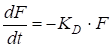(1)

where F is the flake index (in percentage), t is the disintegration time in seconds, and KD is the disintegration kinetic constant function for the operating conditions. The flake index can be expressed by means of the Somerville index (ISV), which represents the non-defibrated paper with respect to the initial total amount of paper to be disintegrated. According to this, Eq. 1 can be expressed as: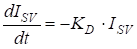(2)

The integration of Eq. 2 between the initial time and t time yields Eq. 3, in which the content of non-disintegrated flakes (ISV) is expressed as function to the initial flake (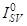) content and the disintegration constant (KD):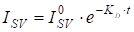(3)

Another way to determine the disintegration constant (KD) is by means of the dissipated energy per unit volume. In general, in a paper suspension, high dissipated energy per unit volume results in a low Somerville index, for a given specific time and suspension consistency. Therefore, one can consider the hypothesis that the number of disintegrated flakes per unit time and per unit mass, is proportional (k) to the instantaneous dissipated power per unit volume (PS) and to the number of flakes per unit mass (N) (Amaral et al. 2000). Equation 4 shows the reduction in the number of flakes as a function of the flake content (N) and the instantaneous power dissipated per unit volume. The corresponding integrated form is given in Eq. 5.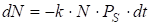(4)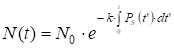(5)

By integrating the instantaneous power per unit volume with respect to time, the energy dissipated per volume unit (SS) is obtained as follows: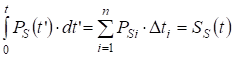(6)

Therefore, Eq. 5 can be expressed as in Eq. 7, or as in Eq. 8 if the flakes are determined by means of the Somerville index: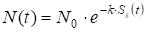(7)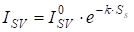(8)

As a result, a linear dependence between ln(ISV) and the energy dissipated per unit volume (SS) is established: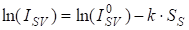(9)

From Eqs. 3 and 7 one can find the value of the disintegration kinetic constant (KD), which is: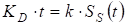(10)

or,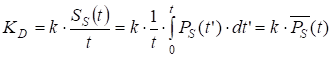(11)

Consequently, the experimental determination of the disintegration kinetic constant is given by measuring the instantaneous power dissipated per unit volume, which tends towards a constant value (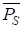), and from the slope k of the linearized expression, Eq. 9.

Modeling of the Disintegration Kinetics

The modeling of the disintegration process makes it possible to compare the experimental results with those obtained after the application of a specific model. As mentioned, disintegration can be expressed as a unit operation having first-order kinetics. If the evolution of disintegration is determined as function of the time by the Somerville index, then the disintegration can be expressed as,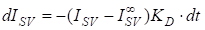(12)

or,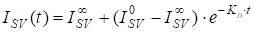(13)

where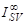represents the limiting value of aggregates that are difficult to break down. It is typically the case for recycled papers that have been treated with wet strength agents.

According to theory (Roux 2001), the first-order kinetic constant is independent of time and results from the product of a sensitivity term (α) by an average shearing stress in the bulk suspension (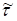) for a given consistency x: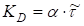(14)

The power dissipated into the fluid per unit volume (PS) is proportional to the mean shearing factor square according to Rayleigh’s dissipation function, where µ is the fluid viscosity: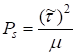(15)

Therefore, the disintegration kinetic constant can be expressed as: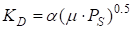(16)

It is worth noting that the inverse of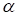has the same dimensions as the dynamic viscosity of the studied fluid.

Assuming that the power dissipated in a fiber suspension is analogous to the power consumed in an equivalent fluid under the same operating conditions (Comiti et al. 2000; Fabri 1999; Puig 2004; Sarquella 2005), it is possible to use the concept of apparent viscosity of a fibrous suspension. This involves the quantification of the whole energy dissipated in the suspension under the form of friction energy. In fibrous suspensions, the apparent viscosity is given by the following equation (Fabry 1999),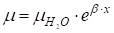(17)

where β is a dimensionless coefficient and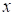the mass fraction expressed in kg of fiber per kg of suspension. The mass fraction (x) is an expression of the consistency, except that it is expressed as a fraction rather than as a percentage. Finally, the term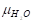is the water viscosity at the disintegration temperature.

If the expression of apparent viscosity is introduced in Eq. 16, this results in a representation of the disintegration kinetic constant as follows: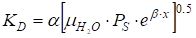(18)

The preceding equation can also be expressed as: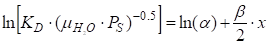(19)

According to Amaral et al. (2000), the first term of Eq. 19 varies linearly with the mass fraction x. The authors also stated thatis function of the mass fraction according to the following relation,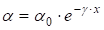(20)

in which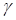is a dimensionless coefficient and (1/α0) is the resistance of the fibrous material to being disintegrated. Therefore, the reorganization of the last two equations gives us,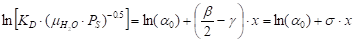(21)

where σ is another dimensionless coefficient. Consequently, the representation of the quantity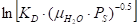as a function of the mass fraction (x) will allow the determination of the numerical constants α0 and σ.

Also from the above expressions, the disintegration kinetic constant can be expressed according to Eq. 22, in which α0 and σ can be analytically determined for a specific raw material, rotor speed, and rotor geometry used in the studied disintegration process.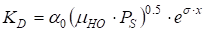(22)

In this work, the kinetics of the disintegration process of coated paper was experimentally determined at different consistencies. The disintegration process was carried out in a neutral pH medium. The experimental results were modeled considering Rayleigh’s dissipation function and both equations (13) and (22).

EXPERIMENTAL

Materials

High quality coated paper from ACONDA PAPER S.A. (Flaçà, Girona, Spain) was used as raw material for determining its disintegration kinetics. The paper was made of two layers, one support-layer (base sheet) composed of fibers and fillers and the coating-layer mainly composed of inorganic fillers. The paper basis weight was 127.5 g/m2, measured according to TAPPI Method T41, and the ash content was 42.5% according to TAPPI T413. The paper ply (support layer) was composed of 45.9 wt% of hardwood kraft pulp, 34.6 wt% of fiber from agroforest residues (which was 87.5% of large fiber and 12.5% of short fiber), and of 19.5 wt% of mineral filler. The coating was composed mainly of mineral filler and binders, and represented 36.5 g/m2 of the paper weight.

Methods

Disintegration process and data acquisition

Coated paper was submitted to the disintegration process by means of a pilot scale pulper (Pulcel cell from Metrotech, France) equipped with a helicoidal rotor, deflectors, and with an effective volume of 20 L. The disintegration was performed at 20 rev/s rotor speed and at a temperature of 50 ºC. The study of the disintegration kinetics for coated paper was completed at 6, 8, 10, 12, 14, 16, and 18% consistency. The initial paper was cut into pieces of 10 x 10 cm, approximately, and was soaked in the water before starting the rotor. The same procedure was followed at each consistency.

The pulper incorporated a network analyzer for the determination of the instant energy and the instant dissipated power. Data were transmitted to a computer by means of a data acquisition system and treated by Lab Windows software. The power dissipated to the suspension (net power) is determined after subtraction the power consumed when running the device in air from the gross power, under identical experimental conditions.

Somerville index determination

After disintegration, the non-disintegrated fiber residual was determined by measuring the Somerville index. The Somerville apparatus is composed of a strainer with slots of 0.15 mm width, and the Somerville index was determined according to TAPPI Useful Method UM242. For this, 25 g of dry pulp was introduced into the Somerville entry box, and the in-water valve was adjusted to 1.225 kg/cm2. The pulp was screened through the Somerville strainer for 20 minutes. The procedure was performed at different disintegration times until the desired defibering residual had been reduced to 0.01%. That means that the limiting Somerville index was taken as 0% in these experimentations.

RESULTS AND DISCUSSION

Table 1 shows the values of the natural logarithm of Somerville index as a function of the disintegration time for every disintegration consistency of recycled coated paper.

Table 1. Values of Ln(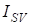%) Obtained at Specified Consistency of Coated Paper Disintegration Performed at a Rotor Speed of 20 rev/s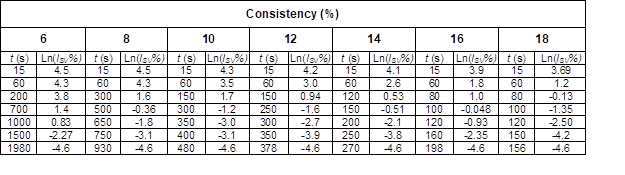On the one hand, it was assumed that the initial percentage of flakes was 100% (non-disintegrated fiber residual of 100% at t=0). On the other hand, the target Somerville index of 0.01% was chosen to represent a good disintegration process. Results from Table 1 demonstrate that as the consistency increased, the disintegration time needed to reach a given defibering residual was decreased. Hence, the disintegration time was reduced from 1980 to 156 seconds when the consistency increased from 6 to 18%.

Taking data from the table, a linear correlation between ln(ISV%) and disintegration time can be established. It was confirmed that disintegration process followed first-order kinetics according to Eq. 3. Therefore, the disintegration kinetic constant (KD) corresponds to the slope of the ensuing linear regression function. The experimental kinetic constant for coated paper disintegration at different consistencies is given in Table 2.

Table 2. Linear Regression Equation, Correlation Coefficient and Experimental Kinetic Constant for Coated Paper Disintegration at Different Consistencies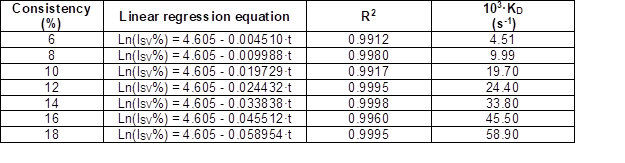A good correlation was established between the Somerville index and the disintegration time at each consistency considered. It was confirmed that the disintegration kinetic constant KDwas an increasing function of the mass fraction x. In the present case, the kinetic constant was multiplied by 13 times when the consistency went up from 6 to 18%.

In order to model the disintegration behavior of coated paper, the term ln[KDH2O·PS)-0,5] in Eq. 21 was determined, knowing the experimental kinetic constant, the measurement of the dissipated power per volume unit (PS), and the water viscosity at 50 ºC (0.547·10-3 Pa·s). The obtained values are presented in Table 3.

Table 3. Values of Ln[KDH2O·PS)-0,5] at Each Consistency of the Disintegration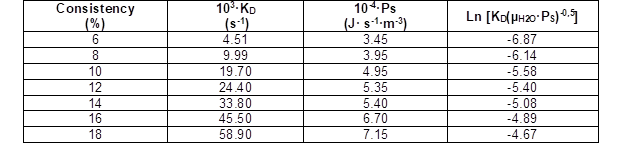Afterwards, the graphic depiction in Fig. 1 confirms a linear dependency between the term ln[KDH2O·PS)-0.5] and the consistency, as indicated by Eq. 21. The linear function obtained for the disintegration of coated paper at the studied rotor speed and rotor geometry was ln[KDH2O·Ps)-0.5] = 0.1717 · x – 7.5786, for which the coefficient of determination was R2=0.93.

From the previous expression, the parameters α0 and σ from Eq. 21 can be determined. In the present case, the deduced α0 and σ values were 5.128·10-4 (Pa.s)-1 and 0.1717, respectively. The reciprocal of the numerical constant α0 is an indicator of the resistance of the pulp to be disintegrated. In our case, (1/α0) was equal to 1950 Pa.s; this numerical value is nearly identical to the 1940 Pa.s found by (Roux 2001) for the disintegration of a mixed of waste papers. The experimentations proposed in this present article are consistent with the previous findings.

Subsequently, the analytical expression for the Rayleigh’s dissipation function, to describe the disintegration kinetic constant of coated paper as function of the consistency was: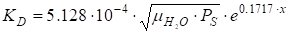(23)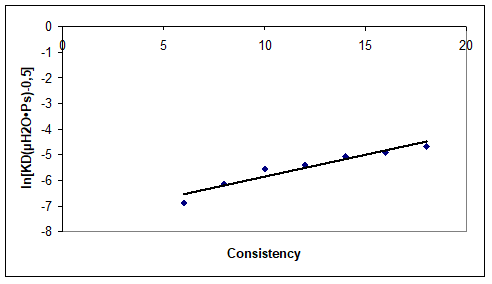Fig. 1. Evolution of ln[KDH2O·Ps)-0.5] with the consistency of coated paper disintegration

The application of the Eq. 23 to the obtained experimental values provides a modeled kinetic constant for the disintegration of coated paper, under the specific rotor speed and geometry. Table 4 gives a comparison between the experimental disintegration kinetic constant and the modeled disintegration kinetic constant, deduced from the Rayleigh’s dissipation function.

Table 4. Comparison between the Disintegration Kinetic Constant Determined Experimentally and the Disintegration Kinetic Constant Deduced from Rayleigh’s Dissipation Function for Coated Paper (Eq. 23).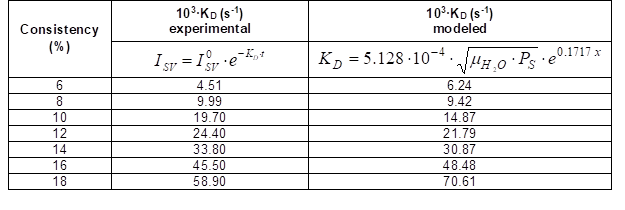The graphic depiction of these values is shown in Fig. 2. A good correlation was found between the modeled and the experimental disintegration kinetic constants. The fitting function between the modeled and experimental kinetic constant in this case was: KD modeled = 1.072 · KD experimental, with a coefficient of determination R2=0.95.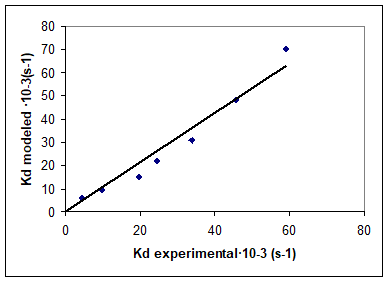Fig. 2. Correlation between the disintegration kinetic constant expressed as Rayleigh’s dissipation function (modeled) and the experimental disintegration kinetic constant

In summary, for the whole range of consistency investigated, the disintegration kinetic constant calculated from Rayleigh’s dissipation function showed a good agreement with the experimental values of the kinetic constant obtained from the evolution of the Somerville index with the disintegration time.

CONCLUSIONS

1. A study of the disintegration kinetics of recovered coated paper was carried out, at different consistency and for a constant rotor speed, geometry and temperature. The evolution of Somerville index (ISV) with disintegration time showed that the un-dispersed residual decreased with increasing consistency, so that disintegration time decreased with increasing consistency.
2. The defiberization process follows first-order kinetics, from which it is possible to obtain the disintegration kinetic constant (KD) in various ways. In the present case the experimental determination of disintegration kinetic constant was obtained from the linear dependency between the logarithm of the Somerville index and the disintegration time. The disintegration kinetic constant is an increasing function of the consistency.
3. The disintegration kinetics was modeled by means of the Rayleigh’s dissipation function. It was confirmed that the disintegration kinetic constant can be expressed as a function of the parameters from Rayleigh’s dissipation function, as obtained from the experimental data, indicating a good correlation between the experimentally derived and the modeled disintegration kinetic constants. This important result was obtained through experimental investigations from the low consistency 6 to the medium consistency range 18%.

ACKNOWLEDGMENTS

The authors are grateful to ACONDA PAPER S.A. for providing the raw material and supporting the experimental study. The authors also thank to the Spanish Ministry of Education and Science the financial support given by the project CTM2008-06886-C0-02TECNO.

REFERENCES CITED

Amaral, M. E., Renaud, M., and Roux J.-C. (2000). “Cinétique de disintegration des pâtes à papier: Modelisation du phénomène,” Revue ATIP 54(3-4), 76-84.

Bennington, C. P. J. (1998). “Understanding defibering and ink detachment during repulping,” Paper Recycling Challenge, 3, Process Technology, Doshi, M. R., and Dyer, J. M. (eds.), Appleton, WI, 268-282.

Bennington, C. P. J., Smith, J. D., and Sui, O. S. (1998). “The effect of mechanical action on waste paper defibering and ink removal in repulping operations,” Journal of Pulp and Paper Science 24(11), 341-348.

Comiti, J., Maure, E., and Renaud, M. (2000). “Mass transfer in fixed beds: Proposition of a generalized correlation based on an energetic criterion,” Chemical Engineering Science 55, 5545-5554.

Fabry, B. (1999). “Etude de la rhéologie des suspensions fibreuses concentrées dans le but d’améliorer le recyclage des papiers,” PhD Thesis, Institut National Polytechnique de Grenoble, Grenoble, (France).

Mutjé, P., López, A., Puig, J., Vilaseca, F., and Pèlach, M. A. (2001). “Disintegration of waste paper. Determination of the shearing factor,” Ingeniería Química 33(378), 115-119.

Puig, J. (2004). “Evaluation of disintegration process of recycled papers,” PhD Thesis. University of Girona (Spain). ISBN: 84-689-0622-0.

Roux, J. C. (2001). “Stock preparation Part 1 – Pulp treatment processes,” 12th Fundamental Research Symposium, 17th-21st September, Oxford (UK), pp. 19-80.

Sarquella, P. (2005) “Physico-chemical aspects in deinking of magazine paper by means of cationic surfactants,” PhD Thesis. University of Girona (Spain). ISBN: 84-689-2574-8.

Savolainen, A., Jussita, T., and Nikula, S. (1991). “Defibering and specific energy consumption in bale pulpers,” Tappi Journal 44(11), 147-153.

Vilaseca, F., Gou, M., Pèlach, M. A., and Mutjé, P. (2000). “Energy consumption and quality of defiberization during the disintegration of waste papers,” Investigación y Técnica del Papel37(145), 423-438.

Article submitted: February 22, 2011; Peer review completed: March 21, 2011; Revised version received and accepted: March 25, 2011; Published: March 28, 2011.